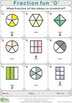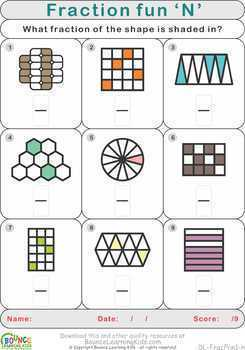# Fraction fun (14 distance learning worksheets for Numeracy)Subject
Resource Type
File Type

Zip

(8 MB|14 pages)
Standards
Also included in:
1. A bundle of products specifically dedicated to fractions & division.Bundle includes a whopping 320 sheets fractions & division sheets from the following products:Fraction codes (36 self-guided fraction games)Fraction codes is a fun series of worksheets to help with recognising and understan
\$29.65
\$19.95
Save \$9.70
• Product Description
• Standards

Making fractions easy and fun with this wonderful series of worksheets that present basic fraction problems is visual form. Depending on the worksheet, the numerator, denominator or both need to be determined based on the visual representation of the fraction. The worksheets start out simple but become progressively more challenging.

Suitable for printing in B/W as well as colour.

Ideal for distance learning - no prep required!

Each worksheet is presented as an individual JPG but there is also a single PDF that contains all the worksheets together, so you have the best of both worlds to choose from.

Free TPT credits:

Go to the My Purchases page and find the Provide Feedback button. Use that to leave a rating/comment. Each time you do this, TPT will give you credits towards future purchases. As an added bonus, the feedback will help me to improve my products :-)

Half price:

Scroll to the top of this page and click on the green star just below the Bounce Learning Kids store name. You'll be able to get new products for half off in the first 24 hours of release!

FREE products:

More quality Visual Learning Resources from Bounce Learning Kids:

Make sense of problems and persevere in solving them. Mathematically proficient students start by explaining to themselves the meaning of a problem and looking for entry points to its solution. They analyze givens, constraints, relationships, and goals. They make conjectures about the form and meaning of the solution and plan a solution pathway rather than simply jumping into a solution attempt. They consider analogous problems, and try special cases and simpler forms of the original problem in order to gain insight into its solution. They monitor and evaluate their progress and change course if necessary. Older students might, depending on the context of the problem, transform algebraic expressions or change the viewing window on their graphing calculator to get the information they need. Mathematically proficient students can explain correspondences between equations, verbal descriptions, tables, and graphs or draw diagrams of important features and relationships, graph data, and search for regularity or trends. Younger students might rely on using concrete objects or pictures to help conceptualize and solve a problem. Mathematically proficient students check their answers to problems using a different method, and they continually ask themselves, "Does this make sense?" They can understand the approaches of others to solving complex problems and identify correspondences between different approaches.
Express whole numbers as fractions, and recognize fractions that are equivalent to whole numbers. Examples: Express 3 in the form 3 = 3/1; recognize that 6/1 = 6; locate 4/4 and 1 at the same point of a number line diagram.
Recognize and generate simple equivalent fractions, (e.g., 1/2 = 2/4, 4/6 = 2/3). Explain why the fractions are equivalent, e.g., by using a visual fraction model.
Understand two fractions as equivalent (equal) if they are the same size, or the same point on a number line.
Explain equivalence of fractions in special cases, and compare fractions by reasoning about their size.
Total Pages
14 pages
N/A
Teaching Duration
N/A
Report this Resource to TpT
Reported resources will be reviewed by our team. Report this resource to let us know if this resource violates TpT’s content guidelines.